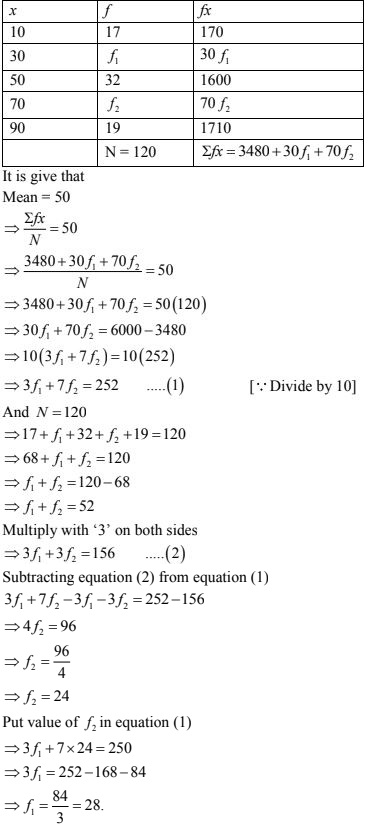# Chapter 24 Measures of Central Tendency RD Sharma Solutions Exercise 24.2 Class 9 MathsChapter Name RD Sharma Chapter 24 Measures of Central Tendency Exercise 24.2 Book Name RD Sharma Mathematics for Class 10 Other Exercises Exercise 24.1Exercise 24.3 Related Study NCERT Solutions for Class 10 Maths

### Exercise 24.2 Solutions

1. Calculate the mean for the following distribution :

 X 5 6 7 8 9 F 4 8 14 11 3
Solution
 X F Fx 5 4 20 6 8 48 7 14 98 8 11 88 9 3 27 N = 40 Σfx = 281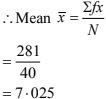2. Find the mean of the following data:
 X 19 21 23 25 27 29 31 F 13 15 16 18 16 15 13
Solution
 X F Fx 19 13 247 21 15 315 23 16 368 25 18 450 27 16 432 29 15 435 31 13 403 N = 106 Σfx = 2650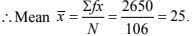3. The mean of the following data is 20.6. Find the value of P.
 x 10 15 P 25 35 F 3 10 25 7 5
Solution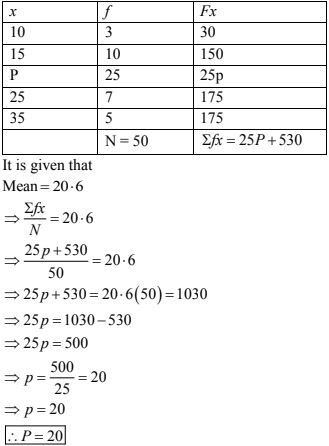4. If the mean of the following data is 15, find P.
 x 5 10 15 20 25 F 6 P 6 10 5
Solution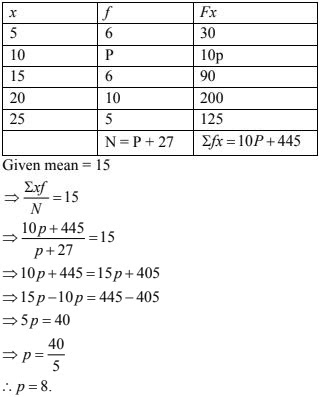5. Find the value of p for the following distribution whose mean is 16.6
 x 8 12 15 P 20 25 30 f 12 16 20 24 16 8 4
Solution6. Find the missing value of p for the following distribution whose mean is 12.58.
 X 5 8 10 12 P 20 25 F 2 5 8 22 7 4 2
Solution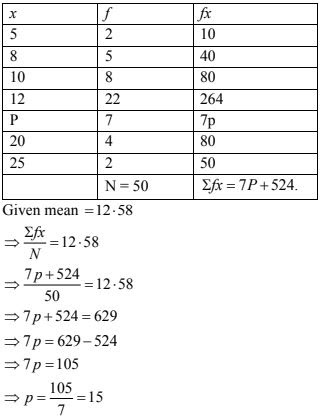7. Find the missing frequency (p) for the following distribution whose mean is 7.68.
 X 3 5 7 9 11 13 F 6 8 15 P 8 4
Solution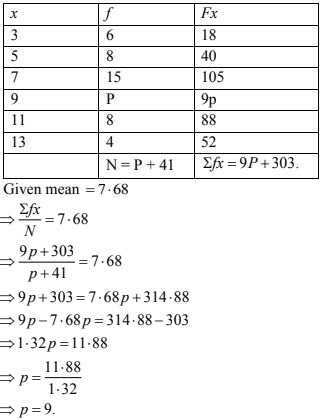8. Find the mean of the following distribution :
 X 10 12 20 25 35 F 3 10 15 7 5
Solution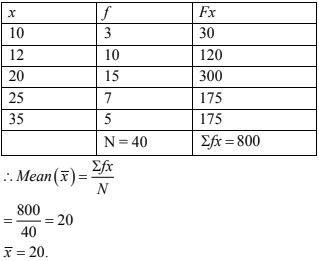9. Candidates of four schools appear in a mathematics test. The data were as follows:
 Schools No. of candidates Average score I 60 75 II 48 80 III NA 55 IV 40 50
If the average score of the candidates of all the four schools is 66, find the number of
candidates that appeared from school III.
Solution
Let no. of candidates appeared from school III = x.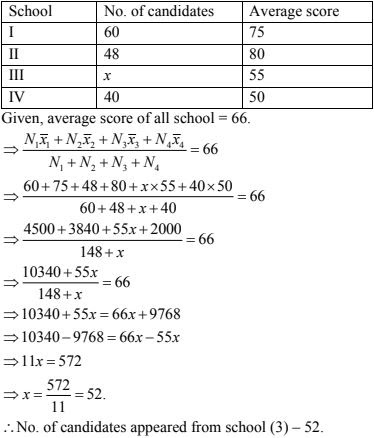10. Five coins were simultaneously tossed 1000 times and at each toss the number of heads were
observed. The number of tosses during which 0, 1, 2, 3, 4 and 5 heads were obtained are shown in the table below. Find the mean number of heads per toss.
 No. of heads per toss No. of tosses 0 38 1 144 2 342 3 287 4 164 5 25 Total 1000
Solution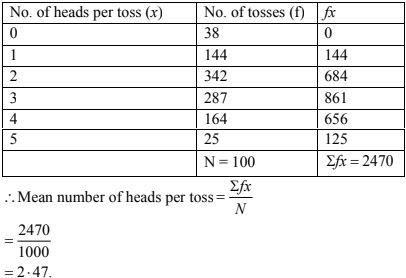11. Find the missing frequencies in the following frequency distribution if its known that the mean of the distribution is 50.
 X 10 30 50 70 90 F 17 F1 32 F2 19 Total = 120
Solution